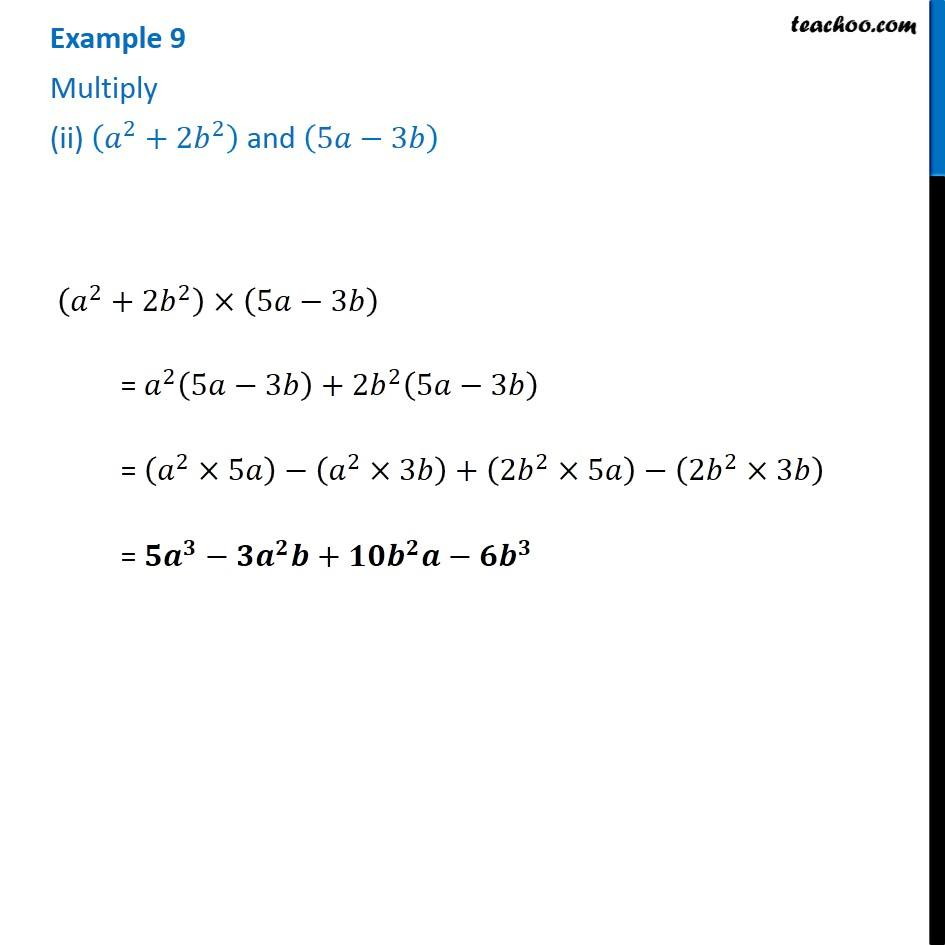Examples

Chapter 8 Class 8 Algebraic Expressions and Identities
Serial order wiseLearn in your speed, with individual attention - Teachoo Maths 1-on-1 Class

### Transcript

Example 9 Multiply (ii) (𝑎^2+2𝑏^2 ) and (5𝑎−3𝑏) (𝑎^2+2𝑏^2 )×(5𝑎−3𝑏) = 𝑎^2 (5𝑎−3𝑏)+2𝑏^2 (5𝑎−3𝑏) = (𝑎^2×5𝑎)−(𝑎^2×3𝑏)+(2𝑏^2×5𝑎)−(2𝑏^2×3𝑏) = 𝟓𝒂^𝟑−𝟑𝒂^𝟐 𝒃+𝟏𝟎𝒃^𝟐 𝒂−𝟔𝒃^𝟑